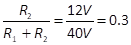Power supply for the grid bias needs to produce DC voltage. Therefore, the power supply is, as usual, composed of a transformer, a rectifier, a reservoir capacitor, a smoothing filter. Finally, there is a voltage divider to obtain the needed voltage. Remember that grid voltage bias is negative with respect to the cathode, which is at ground level. In order to obtain negative voltage, the positive of this power supply is connected to ground and the grid voltage bias is taken from the negative. The complete schema of our power supply for the grid bias is given in Figure 55. Let us analyse how the values of the various components are obtained.

We first compute a simple voltage divider, as the one in Figure 45, then we refine it to obtain the improved circuit shown in Figure 55. In Section 6.1 we determined that the needed grid voltage bias is -12V. Let us assume that the DC voltage obtained after the smoothing filter is -40V. In order to obtain the wanted -12V, the relationship between the two resistors of the voltage divider is as follows:.

Remember also that datasheets specify the maximum allowed impedance between the grid and the cathode of a vacuum tube. In case of the EL84, the maximum impedance between the grid and the cathode is 300K Ohm, when used with fixed bias. In our case, this impedance is the sum of the grid stopper (1K Ohm), the grid leak (200K Ohm), and R2. Accordingly, the value of R2 should be smaller than 100K Ohm. If we chose R1=35K Ohm and R2=15K Ohm, we respect the relationship between the resistors and the limitations on the impedance between grid and cathode as well. The total resistance of the voltage divider is 50K Ohm. With a -40V voltage, the current that flow through the voltage divider is 40V/50K Ohm=0.8mA, which correspond to 32mW dissipated. These very small values allow using a small power transformer.

We can now modify the values computed above, according to the guidelines discussed in Example 26 and Example 27, in Section 5.3.1. In addition, we have to consider that we need two different voltage dividers, for the left and right channel, so the values of R1 and P1 should be doubled.  We obtain the circuit in Figure 55. Value of R2 is brought to 10K Ohm and a potentiometer P2 is connected in series to allow adjusting the grid voltage bias of 25%, roughly from -9V (when P2 resistance is minimum) to -15V (when P2 resistance is maximum). In place of one single resistor R1, we use two resistors of 150K ohm, connected to the two ends of the 10K Ohm potentiometer P1. The circuit with the two resistors R1 and the potentiometer P1 is duplicated twice, for the left and right channel. This allows balancing voltage of the two vacuum tubes in the push-pull stage of 10%, roughly from -11V (when P1 fully turned on one side) to -13V (when P1 fully turned on the other side).